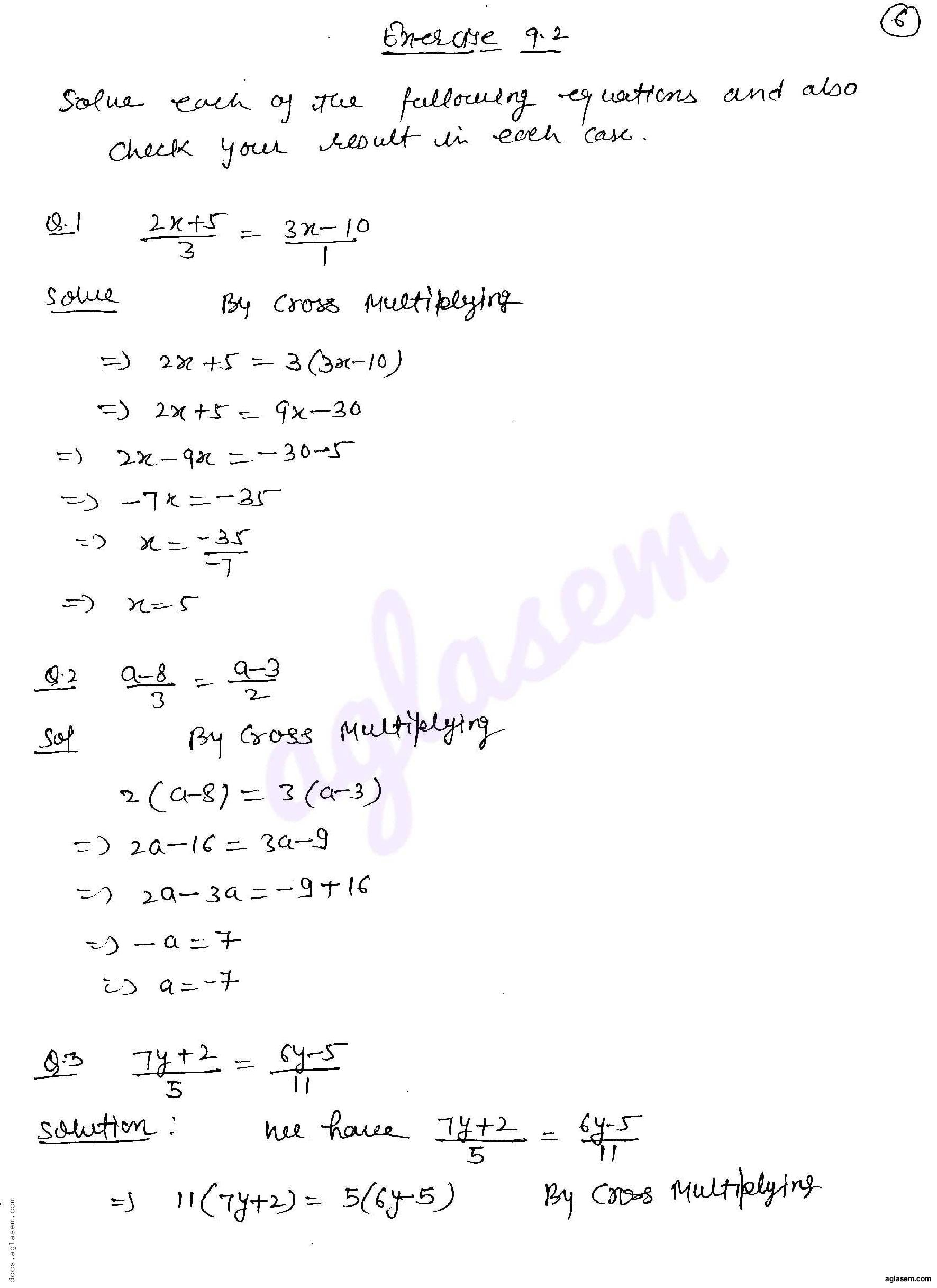aglasem.com

# RD Sharma Solutions Class 8 Chapter 9 Linear Equation in One Variable Exercise 9.2

Get here RD Sharma Solutions Class 8 Maths Chapter 9 Linear Equation in One Variable Exercise 9.2. All answers of Class 8 Maths RD Sharma Book for Chapter 9 Linear Equation in One Variable Exercise 9.2 are given in this PDF. More Detail